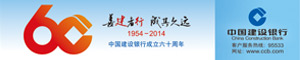2020-03-28 08:51:57来源：阅读：1

Join示例图

# Window Join

``input1.join(input2)    .where(&lt;KeySelector&gt;)      &lt;- input1使用哪个字段作为Key    .equalTo(&lt;KeySelector&gt;)    &lt;- input2使用哪个字段作为Key    .window(&lt;WindowAssigner&gt;)  &lt;- 指定WindowAssigner    [.trigger(&lt;Trigger&gt;)]      &lt;- 指定Trigger（可选）    [.evictor(&lt;Evictor&gt;)]      &lt;- 指定Evictor（可选）    .apply(&lt;JoinFunction&gt;)     &lt;- 指定JoinFunction``

Join的大致流程

``class MyJoinFunction extends JoinFunction[(String, Int), (String, Int), String] {  override def join(input1: (String, Int), input2: (String, Int)): String = {    &#34;input 1 :&#34; + input1._2 + &#34;, input 2 :&#34; + input2._2  }}val input1: DataStream[(String, Int)] = ...val input2: DataStream[(String, Int)] = ...val joinResult = input1.join(input2)      .where(i1 =&gt; i1._1)      .equalTo(i2 =&gt; i2._1)      .window(TumblingProcessingTimeWindows.of(Time.seconds(60)))      .apply(new MyJoinFunction)``

``class MyCoGroupFunction extends CoGroupFunction[(String, Int), (String, Int), String] {  // 这里的类型是Java的Iterable，需要引用 collection.JavaConverters._ 并转成Scala  override def coGroup(input1: lang.Iterable[(String, Int)], input2: lang.Iterable[(String, Int)], out: Collector[String]): Unit = {    input1.asScala.foreach(element =&gt; out.collect(&#34;input1 :&#34; + element.toString()))    input2.asScala.foreach(element =&gt; out.collect(&#34;input2 :&#34; + element.toString()))  }}val input1: DataStream[(String, Int)] = ...val input2: DataStream[(String, Int)] = ...val coGroupResult = input1.coGroup(input2)      .where(i1 =&gt; i1._1)      .equalTo(i2 =&gt; i2._1)      .window(TumblingProcessingTimeWindows.of(Time.seconds(60)))      .apply(new MyCoGroupFunction)``

# Interval Join

Interval Join示意图

``class MyProcessFunction extends ProcessJoinFunction[(String, Long, Int), (String, Long, Int), String] {  override def processElement(input1: (String, Long, Int),                              input2: (String, Long, Int),                              context: ProcessJoinFunction[(String, Long, Int), (String, Long, Int), String]#Context,                              out: Collector[String]): Unit = {    out.collect(&#34;input 1: &#34; + input1.toString() + &#34;, input 2: &#34; + input2.toString)  }}// 数据流有三个字段：（key, 时间戳, 数值）val input1: DataStream[(String, Long, Int)] = ...val input2: DataStream[(String, Long, Int)] = ...val intervalJoinResult = input1.keyBy(_._1)      .intervalJoin(input2.keyBy(_._1))      .between(Time.milliseconds(-5), Time.milliseconds(10))      .process(new MyProcessFunction)``

``val intervalJoinResult = input1.keyBy(_._1)      .intervalJoin(input2.keyBy(_._1))      .between(Time.milliseconds(-5), Time.milliseconds(10))      .upperBoundExclusive()      .lowerBoundExclusive()      .process(new MyProcessFunction)``

Interval Join内部是用缓存来存储所有数据的，因此需要注意缓存数据不能太大，以免对内存造成绝大压力。